# 「MPC-Mike Rosulek 」：Oblivious Transfer and Extension

1. Precomputation: can compute OTs even before you know your input!
2. OT extension: 128 OTs suffice for everything.

OT在多方安全计算中扮演着重要的角色，但OT的实际开销往往很大，因为他不可能使用廉价的加密方法来实现[ImpagliazzoRudich89]。因此在这篇文章中，会介绍一些前沿的方法来提高OT的效率：离线预计算和OT扩展。

# Offline Precomputation

## Random OT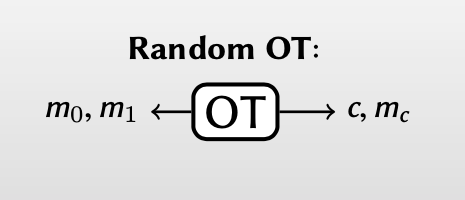Beaver Derandomization Theorem[Beaver91]

There is a cheap protocol that securely evaluates an instance of satandard OT using an instance of random OT.

【Beaver的去随机化理论提出：存在一些高效的协议能将一个random OT 转化为一个standard OT instance.】

## Beaver Derandomization

Beaver去随机化过程分为两个阶段：离线阶段和在线阶段（standard OT)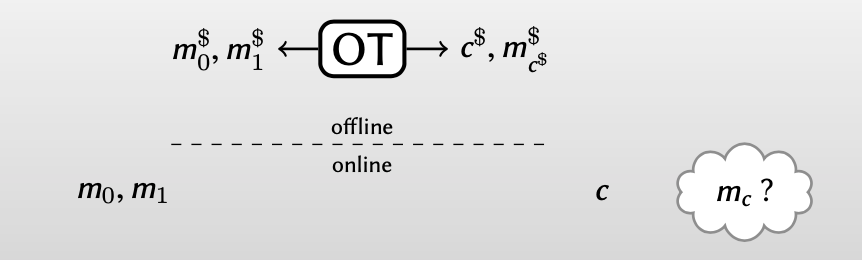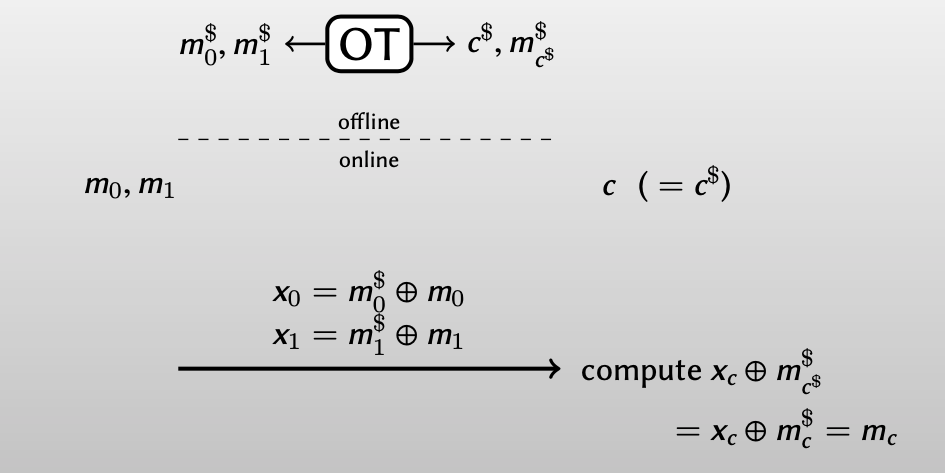• Bob在线时输入： $c\neq c^\$$如果Bob还想用 m_{c^{\}}^\ 直接解密得到 m_c： x_c \oplus m^\_{c^\} =x_c \oplus m^\_{1\oplus c} =m_c ，Alice在加密时 m_0, m_1 时，必须交换其密钥 m_0^\, m_1^\ ：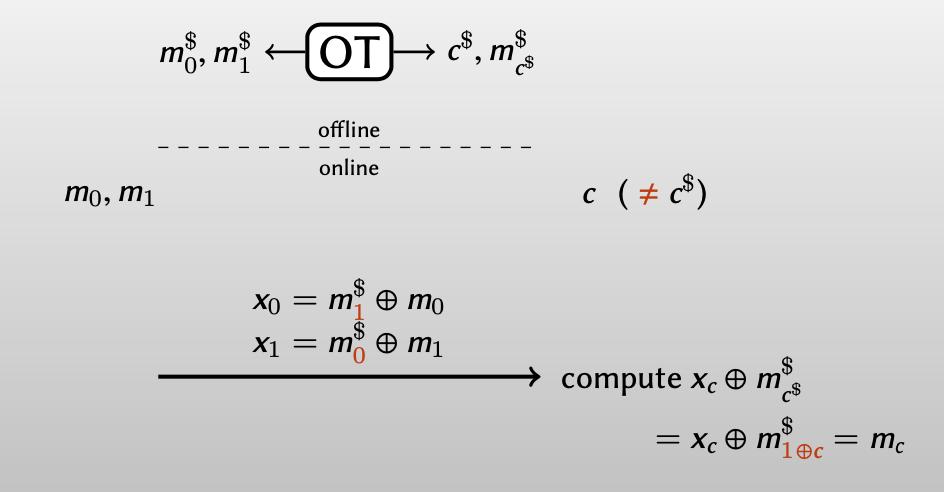为了Bob能得到正确的 m_c ，Bob应该告诉Alice什么时候要交换密钥，什么时候不用交换密钥。 方法就是用一个1-bit one-time pad，Bob 计算出 d = c\oplus c^\$$ ，由此告诉Alice是否需要交换密钥（$d = 0$告诉Alice不需要交换密钥，$d = 1$告诉Alice需要交换密钥），而Alice对$c, c^\$$都一无所知。 因此Beaver的去随机化的完整过程：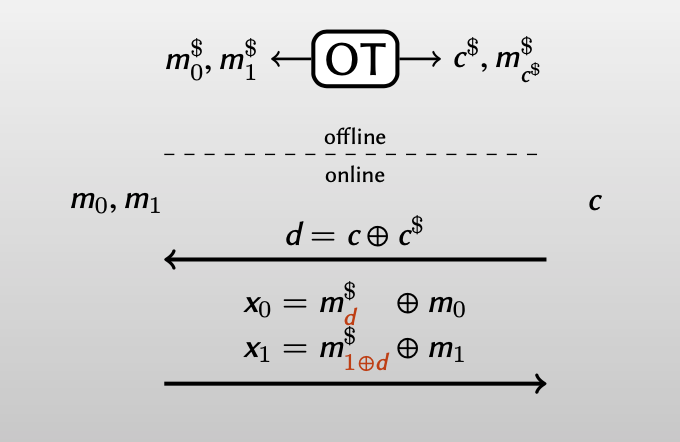1. Bob计算出 d = c\oplus c^\$$ ，发送给Alice，以此告诉Alice是否需要交换密钥 $m_0^\$, m_1^\ 。

2. Alice输入 $m_0, m_1$ ，根据 $d$ 的值选择密钥 $m_0^\$, m_1^\ 来分别加密$m_0, m_1$ :

\begin{align} x_0 &= m_d^\ \oplus m_0 \\ x_1 &= m_{1\oplus d}^\ \oplus m_1 \end{align}

再将其发送给Bob

3. Bob用唯一已知的密钥 $m_{c^{\$}}^\来解密得到 $m_c$： $x_c \oplus m^\$_{c^\$} =x_c \oplus m^\$_{1\oplus c} =m_c$，而对$m_{1-c}$一无所知。 最后分析一下Beaver去随机方法的开销：离线阶段生成一个random OT instance和之前的OT开销相同，但在在线阶段，只需要一些简单的异或运算。 # OT Extension ## An Analogy from Encryption 公钥加密（Public-key encryption, PKE）本质上具有不可避免的高昂开销[ImpagliazzoRudich89] ，但是在现实的加密方案中，可以通过混合公钥加密和对称加密的方式，将实现PKE的开销降到最低。 PKE of λ bits + cheap SKE = PKE of N bits • Use (expensive) PKE to encrypt short s 【用PKE来加密共享短密钥，比如DH算法】 • Use (cheap) symmetric-key encryption with key s to encrypt long M 【再将短密钥通过密钥扩展算法获得长密钥，作为对称加密的密钥】 OT本质上也具有不可避免的高昂开销[ImpagliazzoRudich89] ，所以如果将OT与公钥加密类比，是否存在一种混合加密方案，也能实现λ instances of OT + cheap SKE = N instances of OT ## Beaver OT Extension Beaver利用Yao的两方安全计算，提出了一种OT的扩展协议[Beaver96] 实现$\lambda$instances of OT + cheap SKE =$N$instances of OT. Beaver OT扩展协议其实是一个两方安全计算电路：该电路将Alice和Bob的输入作为伪随机的种子，以此生成$n$OT instances，包括输出给Alice的random strings和输出给Bob的choice bits+choice strings。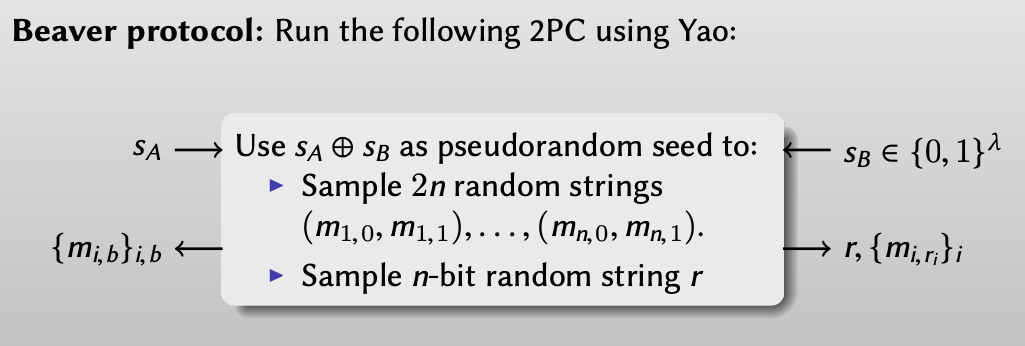该电路的输入位是$\lambda$bit，因此Alice和Bob需要做$\lambda$次OTs，而该电路可以生成$n>>\lambda$OTs，由此实现了$\lambda$instances of OT + cheap SKE =$N$instances of OT. 但是用Yao’s garbled circuits的方法实现Beaver OT扩展协议几乎是不可能的，因为这太复杂了。 ## IKNP Protocol 而Yuval Ishai, Joe Kilian, Kobbi Nissim, Erez Petrank在Crypto 2003年发表的论文：Extending Oblivious Transfers Efficiently，提出了一种可实现、更高效的OT扩展协议，以此实现$\lambda$instances of OT + cheap SKE =$N$instances of OT。 该协议能够用有限的OTs（比如128次OTs）实现足够多次的OTs（比如1 million），下面将详细阐述协议是如何实现的。 ### IKNP Details 1. Bob: 1. has input$r $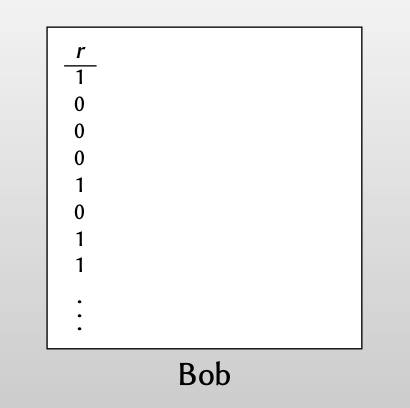2.$\Rightarrow$extend to matrix 【把输入$r$重复多次扩展为矩阵$R$，矩阵的每行$R_i$（ith row of$R$）要么是全1或者全0，而且该矩阵的高是million级别的，宽度可以为128】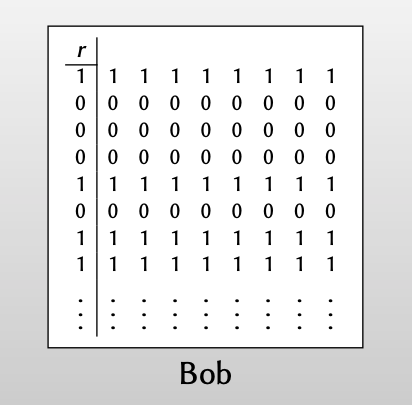3.$\Rightarrow$secret share as ($T, T’$) 【然后对该矩阵$R$做秘密分享，即拆分为两个矩阵$T,T’$】 【秘密分享的策略是随机生成一个矩阵$T$，而$T’=T\oplus R$，观察下图可以看出$R_i = T_i \oplus T_i’$，即两个矩阵的每行要么相同($r=0$)，要么相反($r=1$) 】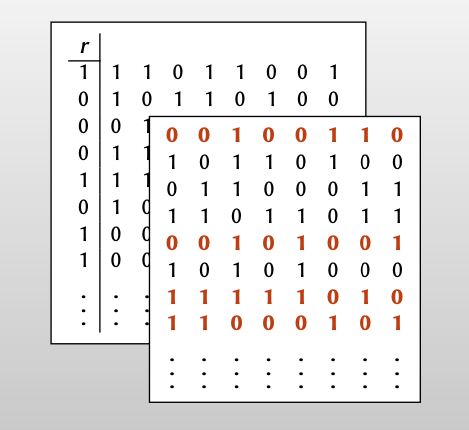2. Alice： 1. chooses random string$s$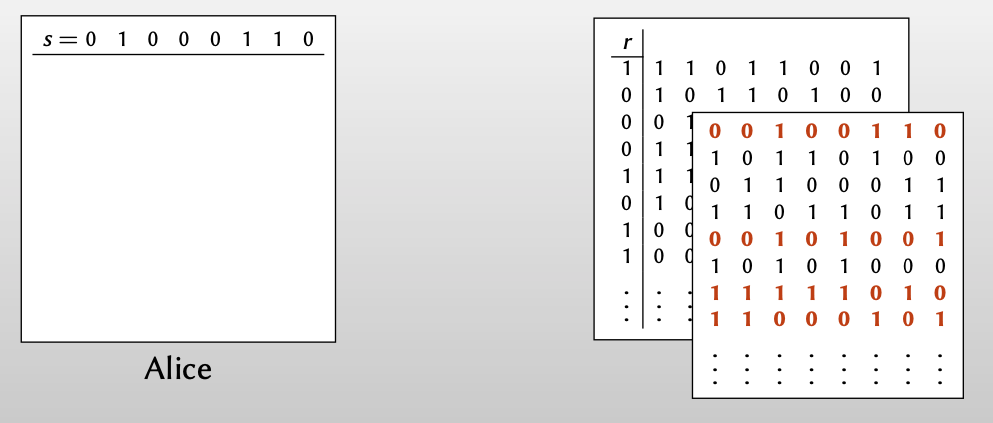2. OT for each column$\Rightarrow$Alice obtains matrix$Q$【从列的角度做OT，Alice每次根据$s$的值从Bob的($T,T’$)选择一列，如果$s_i=0$，从矩阵$T$选择第i列，如果$s_i=1$，从矩阵$T’$选择第i列，得到矩阵$Q$】 【这里的OT中，Bob是sender，Alice是receiver】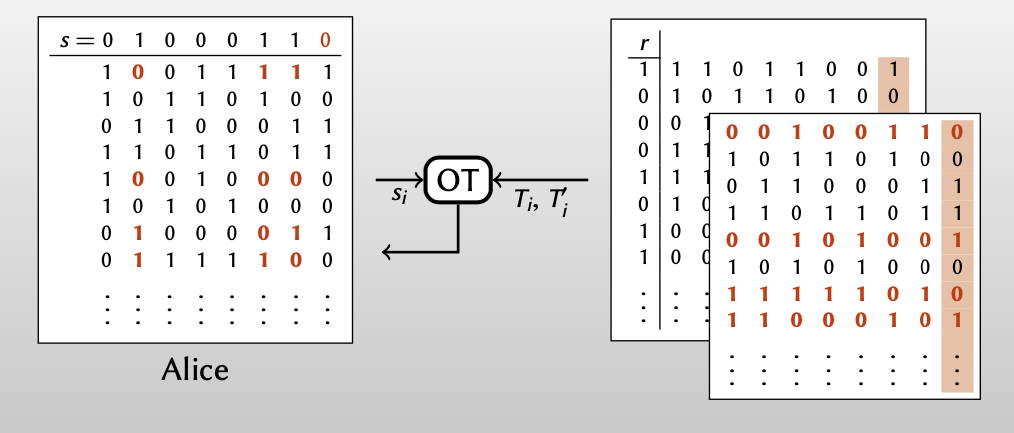观察Alice经过128次OT得到的矩阵$Q$，可以发现Alice得到的矩阵$Q$和Bob拥有的矩阵$T$有如下关系： • Whenever$r_i = 0$, Alice row = Bob row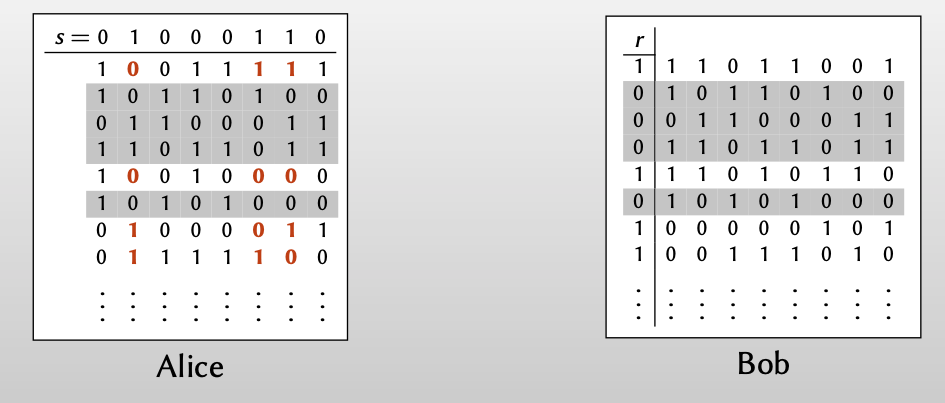• Whenever$r_i =1$, Alice row=Bob row$\oplus s$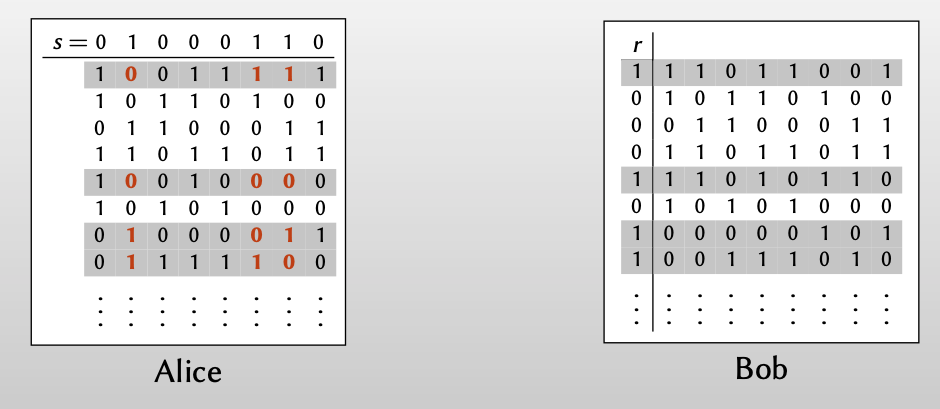3. For every i: Bob knows$t_i$; Alice knows$q_i$and$q_i\oplus s$. 【再从行的角度观察Alice和Bob得到的信息，Alice每次收到$q_i$后，都可以通过和random string$s$异或得到$q_i$和$q_i\oplus s$，而这其中的一个值与Bob知道的$t_i$相同】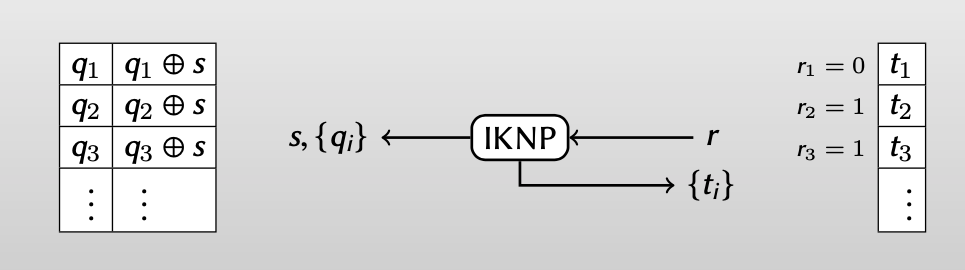【Alice：因为不知道Bob的$r_i$值，所以不知道Bob选择的$t_i$到底是和$q_i$相等还是和$q_i\oplus s$相等】 【Bob：因为不知道Alice的$s$值，所以只知道选择的$t_i$等于$q_i$和$q_i\oplus s$中的一个，而不知道另一个】 但是从Bob视角重写Alice得到的信息，我们知道得到：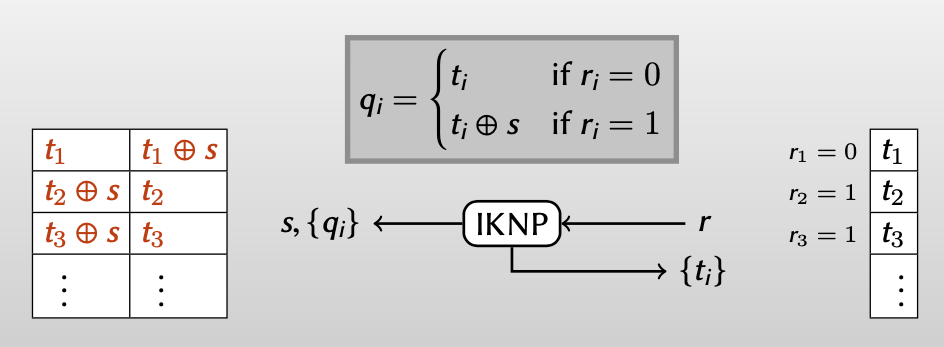•$r_i=0: q_i=t_i$•$r_i=1:q_i=t_i\oplus s$至此，我们几乎已经实现了行角度的OT，Alice有两个值：$q_i$和$q_i\oplus s$，Bob根据$r_i$从中选择得到一个，而对另一个一无所知。 4. Break correlations by applying random oracle 上面我说是“几乎实现了行角度的OT”，为什么是几乎呢？ 因为string s的原因，这些OT instances具有一定的线性相关性。所以为了破坏其中的线性相关性，对这些strings都执行一个任意的密码学函数$H$：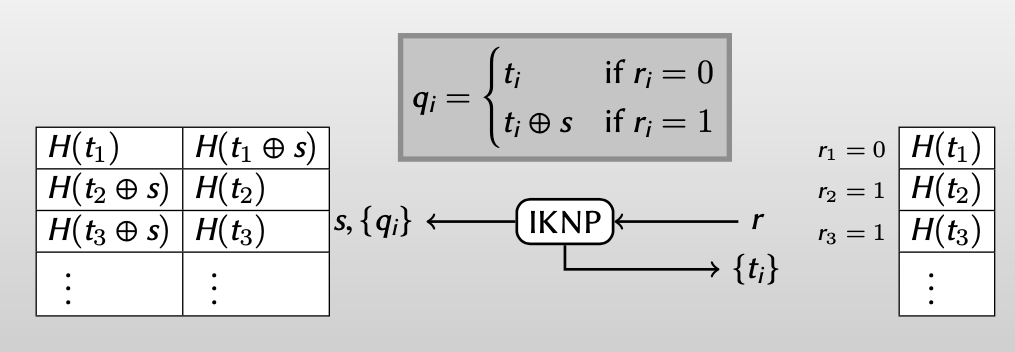5.$\Rightarrow$Random OT instance for each row, using base OT for each column 【由此，通过从列的角度做base OT，得到了足够多的行角度random OT instances】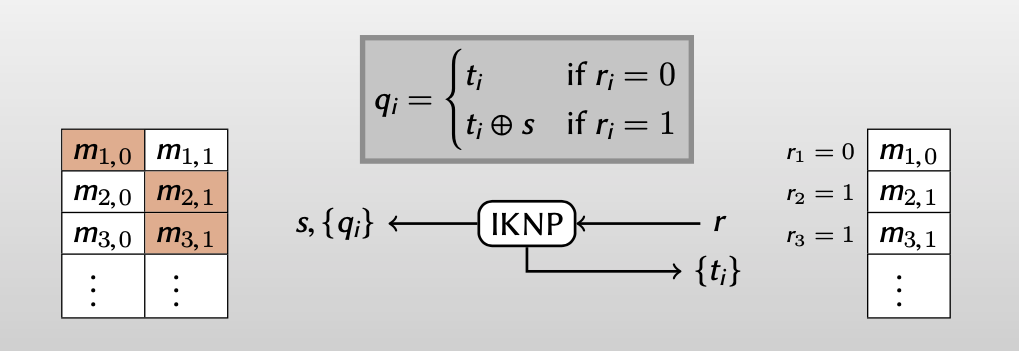最后，总结一下IKNP如何实现$\lambda$instances of OT + cheap SKE =$N$instances of OT。 Bob有一个tall matrix($\lambda$列,$n>>\lambda$行)，Alice从列的角度根据秘密字符串$s$做$\lambda$次base OTs。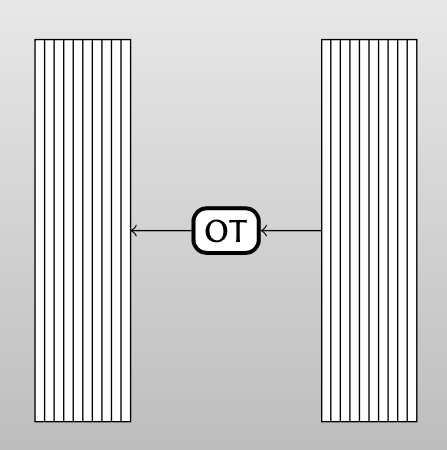最后Alice和Bob得到了行角度的$n$个扩展OTs。### Malicious Bob in IKNP Protocol [KellerOrsiniScholl15] 介绍了如果有恶意的参与方，IKNP受到的威胁 假设Bob是恶意的： 1. Bob： 1. 有一个输入$r $2.$\Rightarrow$对其扩展为矩阵$R$，但是恶意地翻转了$t_2$的第二位bit：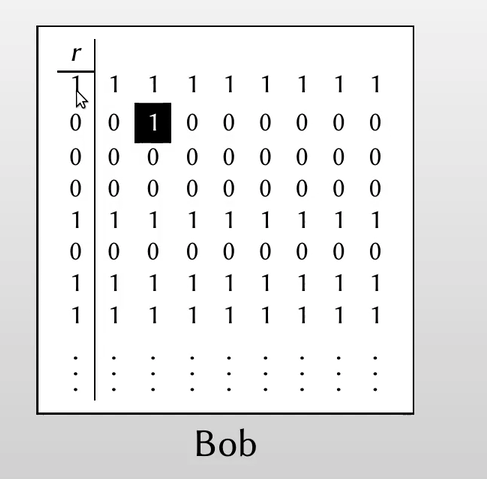3.$\Rightarrow$再做秘密共享为 ($T, T’$) ，同样的，$T’$中也有一位被翻转了：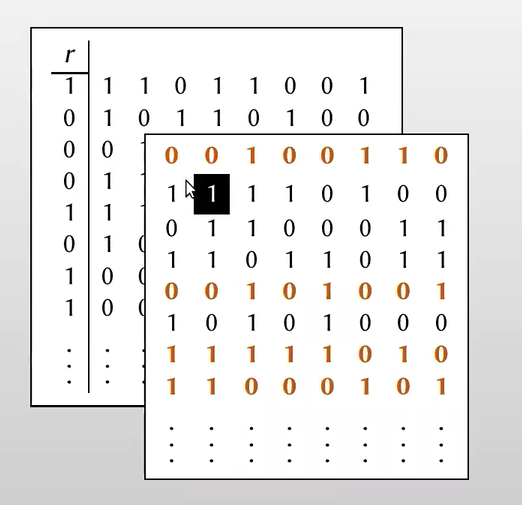2. Alice: 1. 选择一个随机串$s$2. 对每列做OT$\Rightarrow$Alice得到矩阵$Q$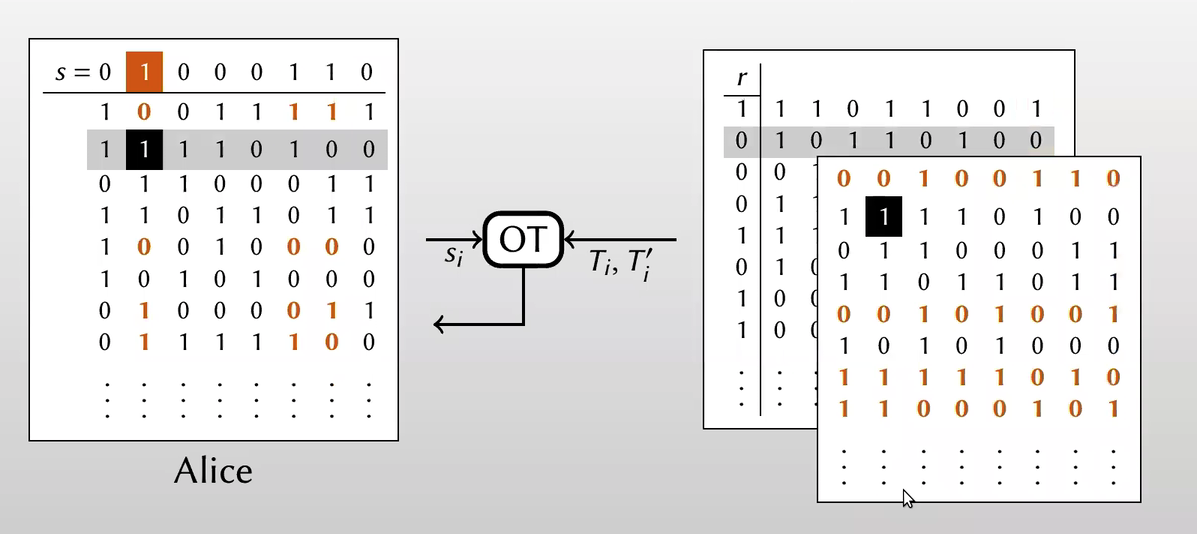因为$r_2=0$，本应该有$q_i = t_i$。但因为Bob恶意翻转了一位，所以Alice得到的$q_i$和$t_i$在第二位不同 3. 所以在Alice和Bob同时使用$H(row)$破坏线性相关性后，Bob会发现得到的$H(row#2)$值不同，Bob就知道Alice选择的随机串中$s_2=1$。 如此以来，Bob每次都恶意翻转某一列的一个bit，就可以学到$s$的所有比特信息。 ### Consistency Check 所以如何保证Bob没有恶意篡改矩阵$R_i$($R$矩阵的第i行)? [KellerOrsiniScholl15] 提出了一种一致性检验方法来检测Bob是否恶意篡改了矩阵。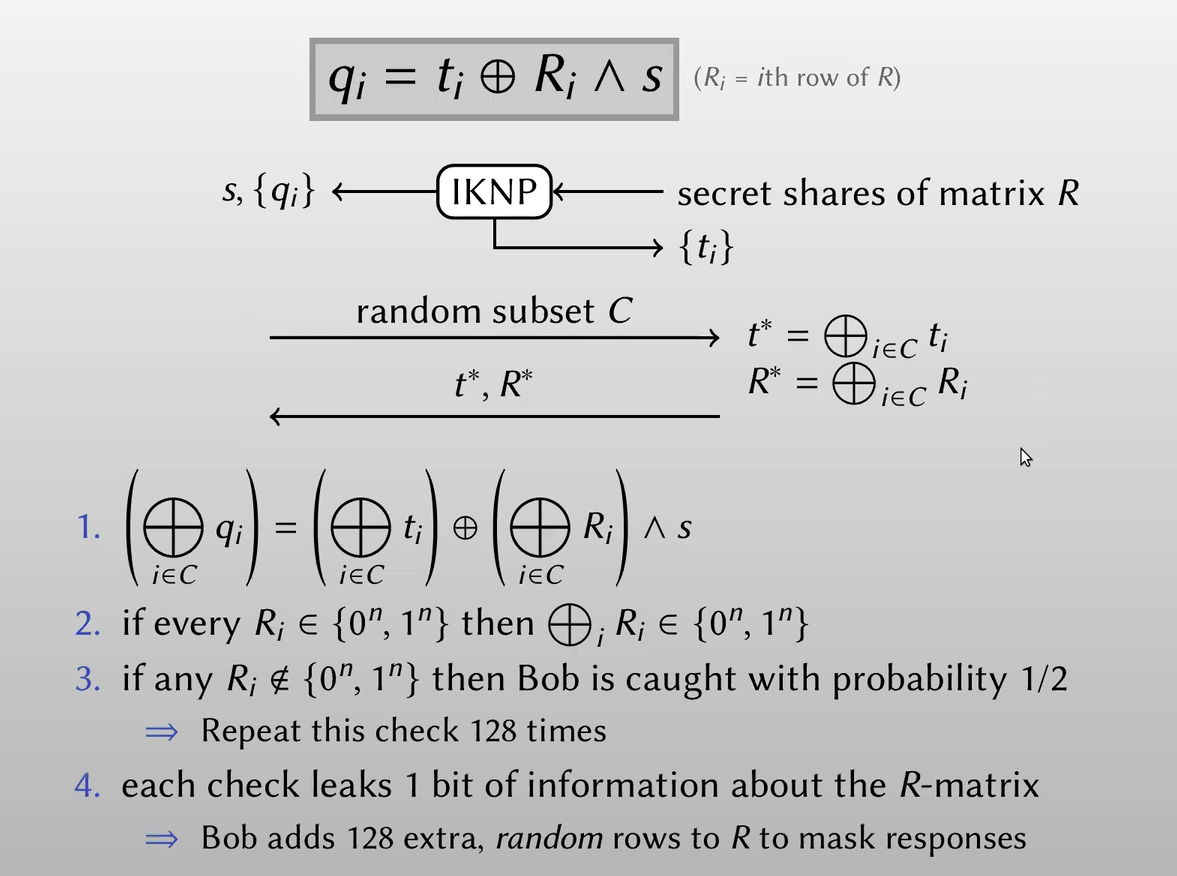Alice在执行IKNP中通过多次检验上述方程的一致性，来检测Bob是否恶意篡改了扩展矩阵。 同时，为了保护扩展矩阵的位信息，Bob也使用一个随机矩阵来加密$R^*$。 IKNP可以通过上述一致性检验来实现malicious security，而引入的额外开销很小。 ## Generalizing IKNP IKNP协议生成了许多二选一的OT instances，这里将介绍如何对IKNP协议一般化，以生成多选一的OT instances。 再次回顾一下IKNP的做法： 1. Bob有一个选择串$r$2. 扩展为矩阵$R$，矩阵的每行是全0或全1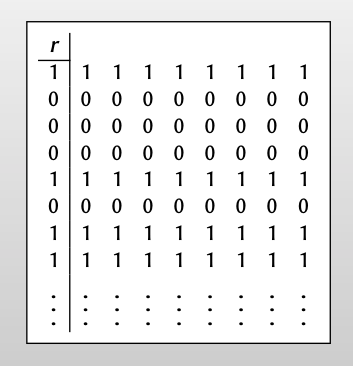3. 再对矩阵R做秘密分享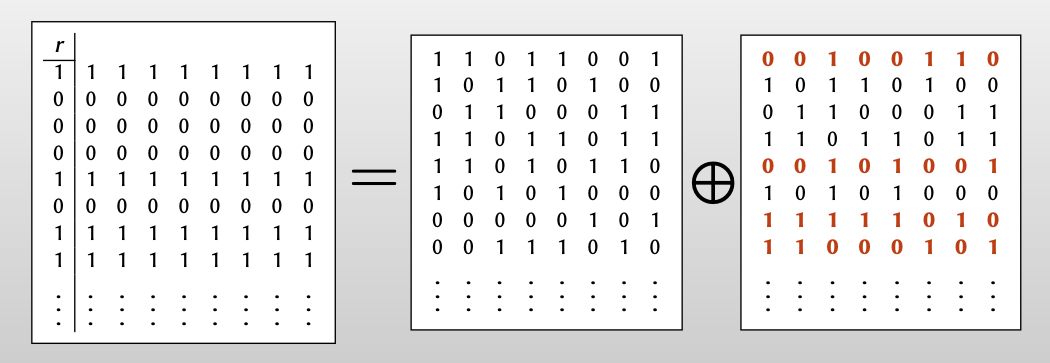从行的角度看矩阵扩展，把0 编码为 000... ，把1 编码为111... 。所以可以把矩阵扩展看作对$r$的bit的一种编码方式（repetition code）。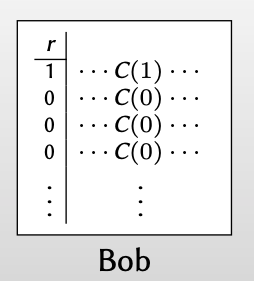因此，一般化IKNP的核心思想就是：用具有差错校正的编码方式对$r$的bit进行编码。 ### Coding view of IKNP 现在，我们从编码的方式看IKNP。 1. Bob: 1. Bob 有一个输入$r$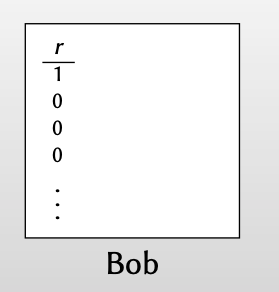2. 对$r$的比特进行编码：3. 在这种编码方式下进行秘密分享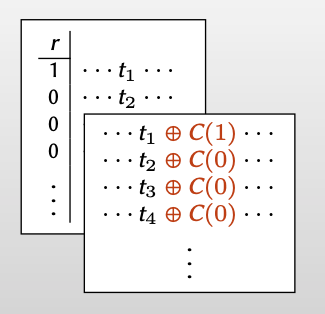2. Alice 对每列做OT，得到矩阵$Q$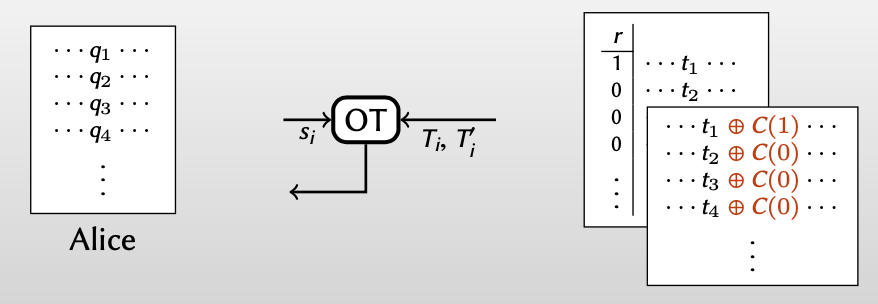Bob得到的$t_i$和Alice得到的$q_i$有如下关系：$t_i = q_i\oplus C(r_i)\wedge s$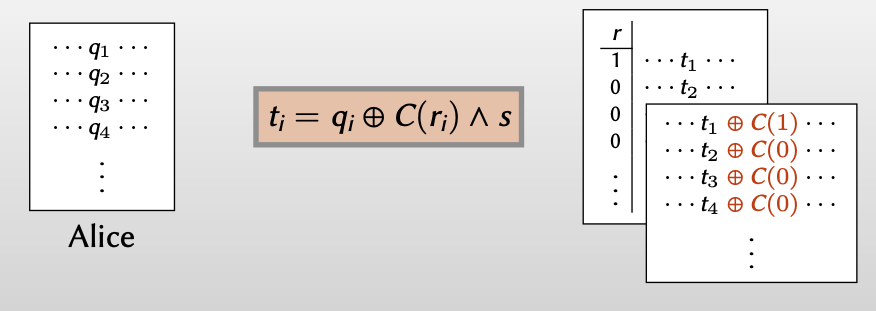如果是在编码$C(0)=000…, C(1)=111…$下： •$r_i=0 \Rightarrow t_i = q_i\oplus (000…)\wedge s=q_i$•$r_i=1 \Rightarrow t_i=q_i\oplus (111…)\wedge s=q_i\oplus s$3. For every i: Bob knows$t_i$; Alice knows$q_i\oplus C(0)\wedge s$and$q_i\oplus C(1)\wedge s$【此时Alice知道$q_i\oplus C(0)\wedge s$和$q_i\oplus C(1)\wedge s$，Bob知道$t_i$】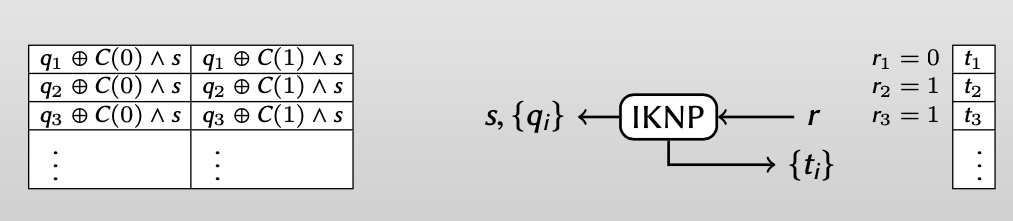• 从Bob的视角重写Alice得到的$q_i=t_i\oplus C(r_i)\wedge s$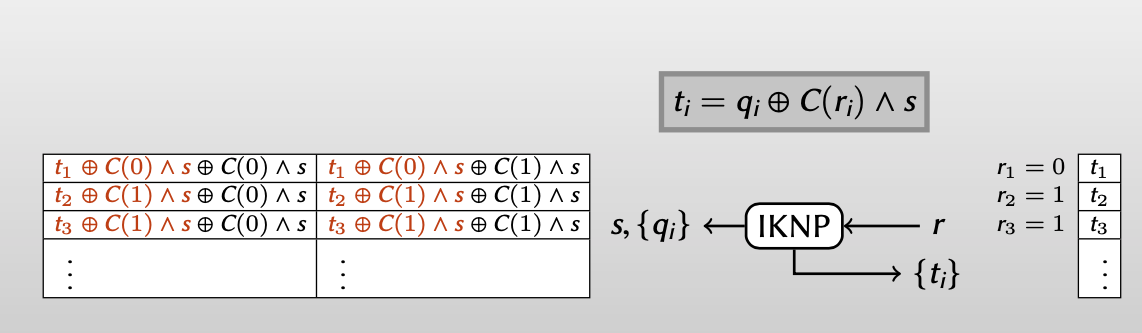• 当$C$是一种线性编码时，即满足:$[C(a)\wedge s]\oplus [C(b)\wedge s]=C(a\oplus b)\wedge s$and$C(0)\wedge s = 000…$得到：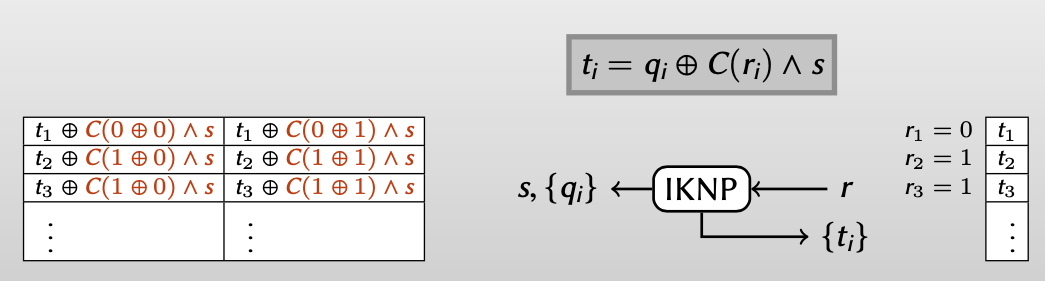化简：4. Use random oracle to destroy correlations 【再破坏其线性相关性】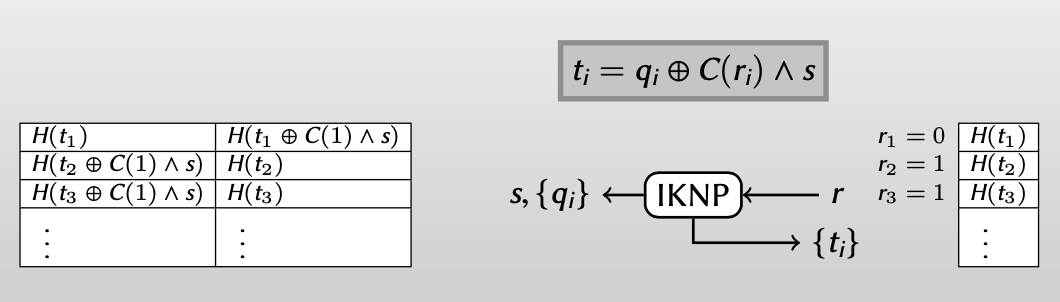### Generalizing IKNP 刚刚我们考虑的是只对$\{0, 1\}$编码，即：$C:\{0, 1\}\rightarrow \{0, 1\}^k$编码。 现在考虑编码更多的bits，即$C:\{0, 1\}^3\rightarrow \{0, 1\}^k$，同样地，$C$是线性编码。 同样的运行IKNP： 1. For every i: Alice can compute 8 (things) 【之前Alice是可以计算$q_i\oplus C(0)\wedge s$和$q_i\oplus C(1)\wedge s$】 现在是对$\{0, 1\}^3$编码，就可以计算：$q_i\oplus C(000)\wedge s, q_i\oplus C(001)\wedge s, \cdots,q_i\oplus C(111)\wedge s$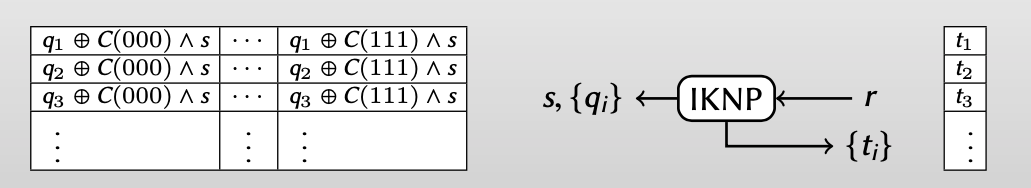• 同样的，从Bob的角度重写Alice得到的值$q_i=t_i\oplus C(r_i)\wedge s$• 并且$C$是线性编码，满足$[C(a)\wedge s]\oplus [C(b)\wedge s]=C(a\oplus b)\wedge s$and$C(0)\wedge s = 000…$，化简后：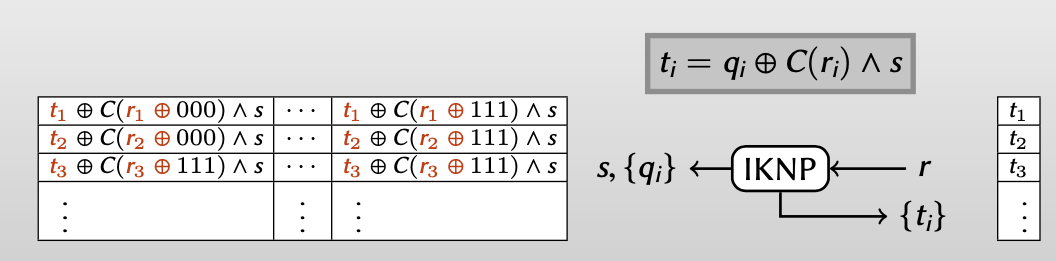对上述Alice计算出的8个串，Bob只知道其中的一个$t_i$，而这个$t_i$等于Alice知道的第几个串，这取决于Bob的选择的$r_i$，使得$C(r_i\oplus \cdots)=000…$。 2. In the random oracle model:$H(t_1 \oplus c_1 \wedge s),…H(t_n \oplus c_n \wedge s)$pseudorandom if all$c_i$have Hamming weight$\ge \lambda$【在破坏线性相关性时，只有当所有$c_i$的汉明重量（Hamming weight）$\ge\lambda$时，$H(\cdot)$和随机串才具有不可区分性】 汉明重量（Hamming weight）:汉明重量是一串符号中非0符号的个数。 汉明距离（Hamming distance）：两个字符串对应位置的不同字符的个数。 就此，我们介绍了扩展的IKNP协议，[KolesnikovKumaresan13] 提到使用这样的编码：$C:\{0, 1\}^l\rightarrow \{0, 1\}^k$(编码字符串的汉明距离$\ge \lambda$) 执行$k$次base OT，就能实现从$2^l$中选一个的OT扩展(1-out-of-$2^l$OT extension)。 • 1-out-of-256 OT[KolesnikovKumaresan13] : Walsh-Hadamard code$C:\{0, 1\}^8\rightarrow \{0, 1\}^k$(min. dist. 128) • 1-out-of-$2^{76}$OT[OrruOrsiniScholl16] : BCH code$C:\{0, 1\}^{76}\rightarrow \{0, 1\}^{512}$(min. dist. 171) • 1-out-of-∞ OT[KolesnikovKumaresanRosulekTrieu16] 这篇文章提出，对于任意的伪随机编码$C:\{0, 1\}^*\rightarrow \{0, 1\}^{～480}$，只需要满足最小的hamming dist.，而不用要求编码$C\$ 的线性性质，就可以实现1-out-of-∞ OT。

「MPC-Mike Rosulek 」：Oblivious Transfer and Extension

https://f7ed.com/2021/07/07/MPC3-OT/

f1ed

2021-07-07

2022-06-28